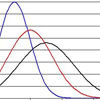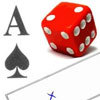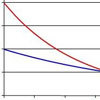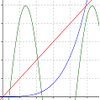Search by Topic

Resources tagged with Probability density functions similar to Scale Invariance:

Filter by: Content type:
Age range:
Challenge level:

There are 9 results

Broad Topics > Advanced Probability and Statistics > Probability density functionsScale Invariance

Age 16 to 18 Challenge Level:

By exploring the concept of scale invariance, find the probability that a random piece of real data begins with a 1.Pdf Stories

Age 16 to 18 Challenge Level:

Invent scenarios which would give rise to these probability density functions.PDF

Age 16 to 18 Challenge Level:

Given a probability density function find the mean, median and mode of the distribution.Into the Normal Distribution

Age 16 to 18 Challenge Level:

Investigate the normal distributionCircle Pdf

Age 16 to 18 Challenge Level:

What happens if this pdf is the arc of a circle?Distribution Differences

Age 14 to 16 Challenge Level:

How could you compare different situation where something random happens ? What sort of things might be the same ? What might be different ?Into the Exponential Distribution

Age 16 to 18 Challenge Level:

Get into the exponential distribution through an exploration of its pdf.PCDF

Age 16 to 18 Challenge Level:

When can a pdf and a cdf coincide?Exponential Intersection

Age 16 to 18 Challenge Level:

Can the pdfs and cdfs of an exponential distribution intersect?